Pressure losses in the pipes, rate of flow (laminar, turbulent, critical zone), viscosity, calculation of the Reynolds number, influences rate of antifreeze (glycol).
French site
| Home | Thematic | Tables | Programs | Library | Download | Links | Contact | Forum |--

linear pressure losses
 Thematic Quotation Margin Hydraulic Linear pressure loss Local pressure loss Compressible fluids No circular duct Thermal Thermal fluid flow Pipe sizing steam Control valves Orifice plate Pumps Ventilators Gas Fuel gasPressure losses distributed in the pipes

The calculation of the linear pressure loss, that corresponding to the general flow in a rectilinear conduit, is given by the following general formula: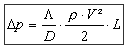• Dp = pressure loss in Pa
• L = friction factor (a number without dimension)
• p = density of water in kg/m3
• V = flow rate in m/s
• D = pipe diameter in m
• L = pipe length in m

The expression above shows that calculations of pressure losses rest entirely on the determination of the coefficient L.

 Flow type

The nature of the type of flow of a fluid is determined by the value of the Reynolds number.

The various types of flows are visualized by the chart of the diagram of Moody using the Reynolds number for the x axis and the factor of friction F for the y axis.

The mode of flow of a fluid is characterized in 3 forms:

 Laminar mode Reynolds number lower than 2000 indicates than the flow is calm and regular Turbulent mode Reynolds number higher than 4000 indicates than the flow is in the form of swirl and of movement. Zone critique Reynolds number located 2000 and 4000 indicates that the flow is unstable between the laminar mode and the turbulent mode.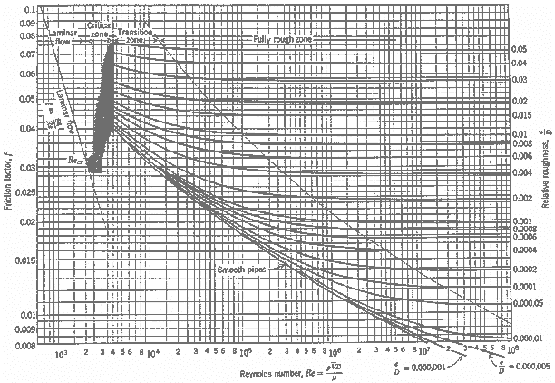Calculation of the Reynolds number

The Reynolds number is no dimensional (thus without units). It combines 3 significant characteristics of the flow and the fluid: speed, density and viscosity. The diameter is necessary to make the number no dimensional. One calls the diameter the characteristic length. A Reynolds number of 2000 or less indicates a flow in laminar mode while a number of 4000 where more a turbulent flow indicates.

The Reynolds number is defined is:

 According to kinematics viscosity According to dynamics viscosity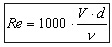V = flow rate in m/s d = pipe diameter in mm v = viscosity of water in mm²/s (or centistokes) (legal System (S.I) in m²/s = 1000000 centistokes or mm²/s) p = density in kg/m3 V =speed in m/s D = hydraulic diameter of the pipe in m µ = dynamic viscosity in Pa.s (or kg/m.s) (kg/m.s = One tenth of a poise = 10 poises)

Reynolds number is inversely proportional to kinematics viscosity.

The viscosity of a fluid is a characteristic which makes it possible to determine resistance to the movement of the fluid. The higher kinematic viscosity will be and the more difficult it will be to move the fluid in the pipe.

Kinematics viscosity (v is the ratio of dynamic viscosity on the density of the fluid.

 Kinematics viscosity in m2/s kinematics viscosity in mm²/s (or centistokes)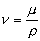v = kinematics viscosity in mm²/s (or centistokes) - (legal system (S.I) in m²/s = 1000000 centistokes) µ = viscosity dynamic of water Pa.s or (kg/m S) p = density of water in kg/m3
 Loss pressure

Laminar flow (Re £ 2000)

In rate of laminar, the nature or the surface quality of the interior walls of the lines does not intervene in the calculation of the pressure loss.

The loss pressure is determined by the following function:• L = friction factor (a number without dimension)
• Re = Reynolds number

The laminar flow meets in practice only in the transport and the handling of the viscous fluids, such as the crude oil, fuel oil, oils, etc.

Turbulent flow (Re > 2000)

In the critical zone, i.e. between 2000 and 4000 Reynolds the formula of computation employed will be treated in the manner that in situation of mode of turbulent flow.

In rate of turbulent, the factor of friction is translated by the formula of Colebrook considered as that which translates best the phenomena of flow into turbulent mode.It is noted that this formula is in implicit form; consequently search can be done only by successive approaches (iterative calculation)

With:

• L = friction factor (a number without dimension)
• D = pressure loss coefficient.
• k = index of roughness of the pipe.
• d = pipe diameter in mm.
• Re = Reynolds number.
 Usual value index of roughness (k) in mm Nature of interior surface Index roughness K 1 Copper, lead, brass, stainless 0,001 to 0,002 2 PVC pipe 0,0015 3 Stainless steel 0,015 4 Steel commercial pipe 0,045 à 0,09 5 Stretched steel 0,015 6 Weld steel 0,045 7 Galvanized steel 0,15 8 Rusted steel 0,1 to 1 9 New cast iron 0,25 to 0,8 10 Worn cast iron 0,8 to 1,5 11 Rusty cast iron 1,5 to 2,5 12 Sheet or asphalted cast iron 0,01 to 0,015 13 Smoothed cement 0,3 14 Ordinary concrete 1 15 Coarse concrete 5 16 Well planed wood 5 17 Ordinary wood 1
 Influence rate of antifreeze (glycol)

In the case of an addition of antifreeze (glycol) to water, kinematics viscosity (into centistokes) varies in the following way: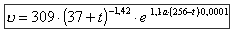• t = temperature at 0°C
• a = percentage of glycol

Last update: